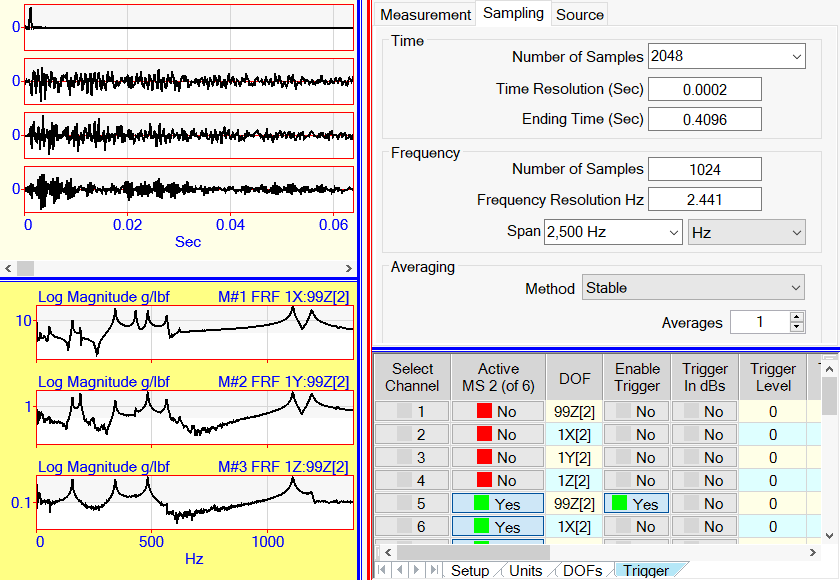# Sampling Tab

The Acquisition window acquires finite length blocks of time domain data from all active channels of the connected front end

• All acquired time domain waveforms are displayed in the upper graphics area in the Acquisition window.

The Sampling tab is used to setup the following data acquisition parameters ;

• The Time Number of Samples in each acquired time waveform block off data.

• The Frequency Span (1/2 of the sampling rate) of the time waveforms

All other parameters in the Time and Frequency groups are calculated from the Time Number of Samples and the Frequency Span.
See the DFT and FFT section in the Signal Processing Commands chapter for details.Acquisition Window Showing the Sampling Tab.

## Averaging

Defines the number of spectrum estimates to be used during the calculation of the calculated measurement functions in the lower graphics area

• For impact testing, no more than 5 averages (5 spectrum estimates) are recommended.

• For shaker testing using random signals, between 25 &100 averages (25 &100 spectrum estimates) are recommended.

## Averaging Method

Stable averaging is the same as summing together all of the spectrum estimates and dividing by the number of estimates.

• The Nth stable average is calculated with the formula,

Stable Average (N) =  (1 / N) x New Spectrum + (1 - (1 / N)) x Stable Average (N-1)

Peak Hold averaging retains in the final spectrum the peak value at each sample among all spectral estimates.

• The Jth sample of the Nth average is determined with the formula,

Peak Hold Average (N,J) = maximum (New Spectrum, Peak Hold Average (N-1,J) )